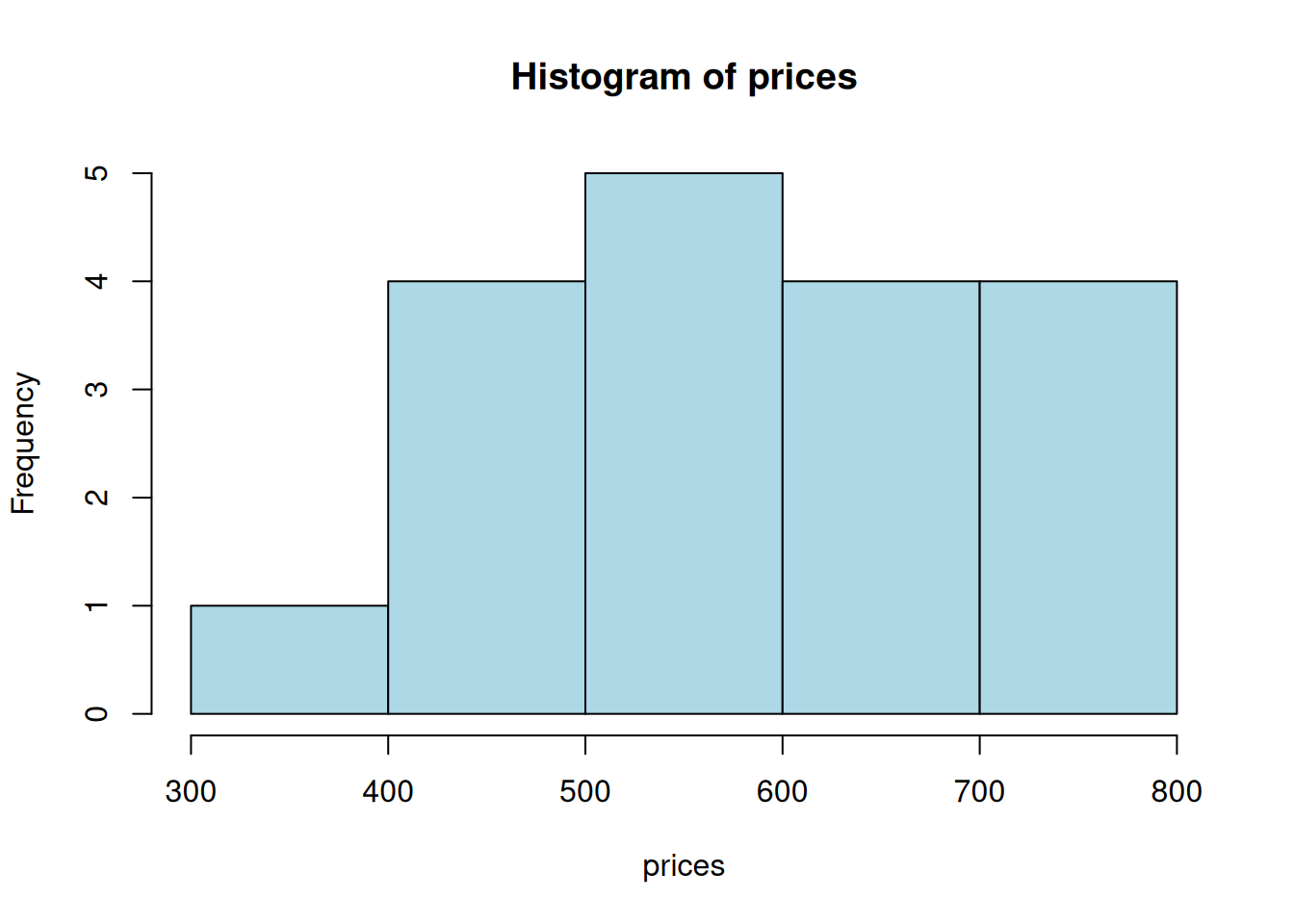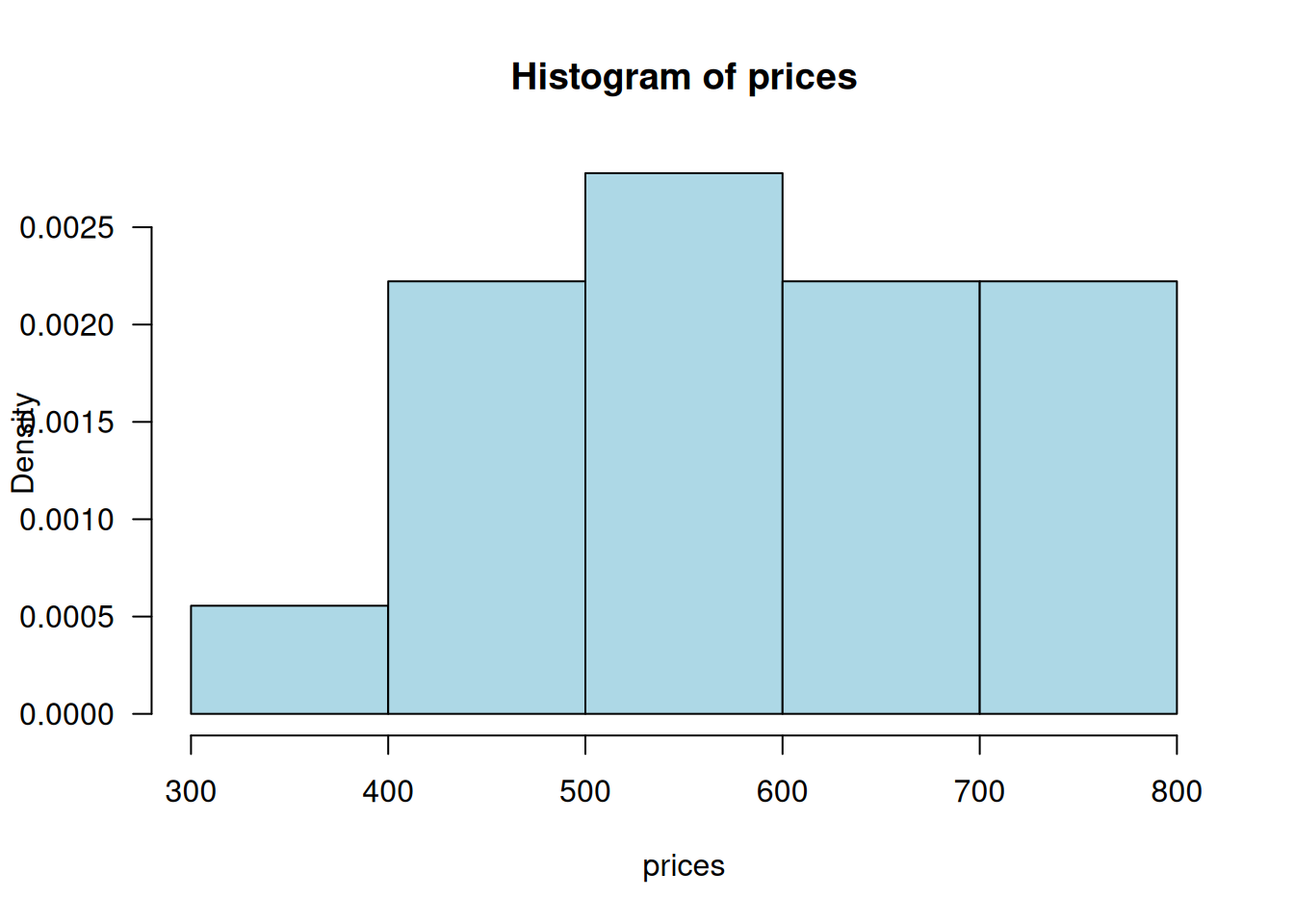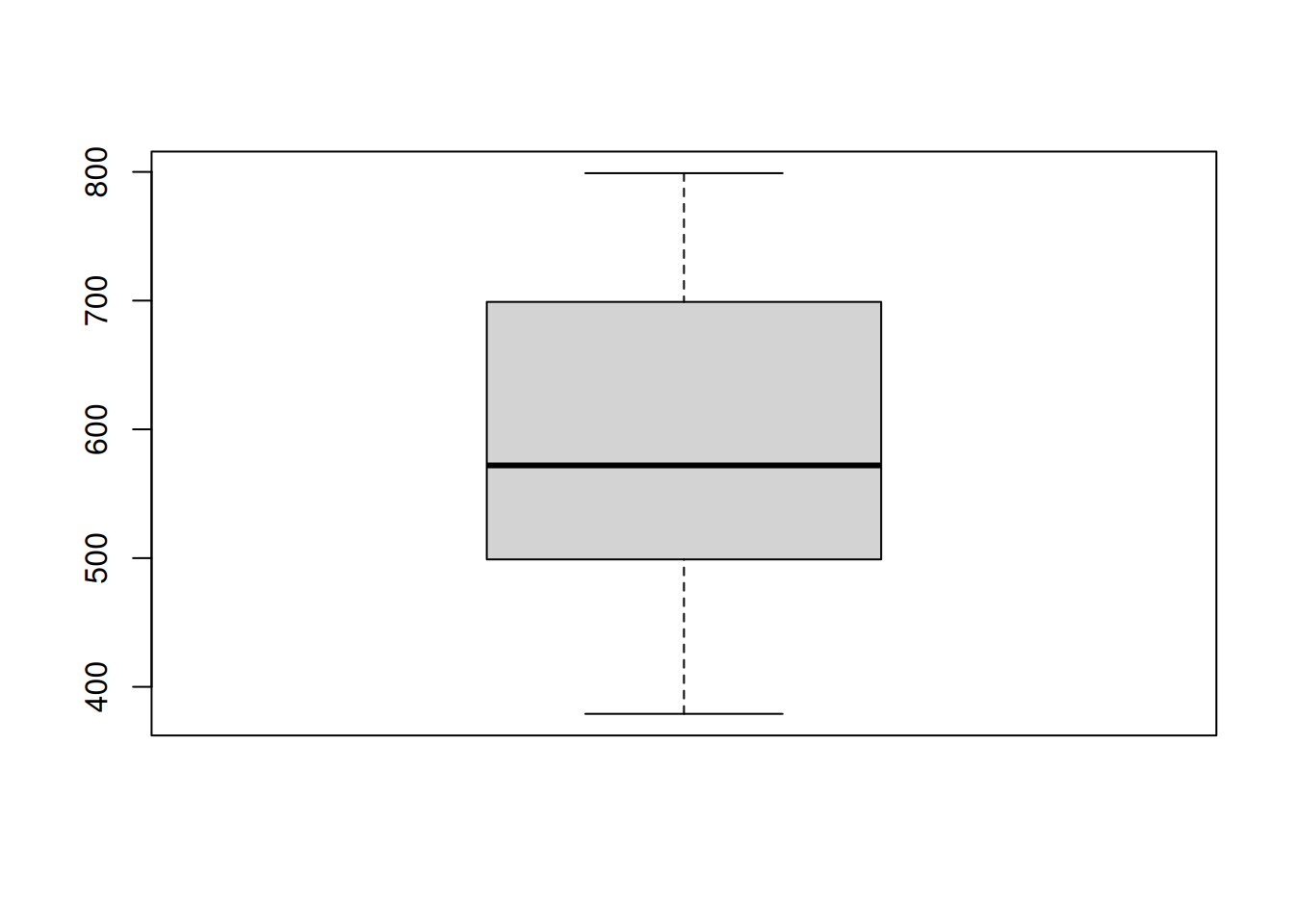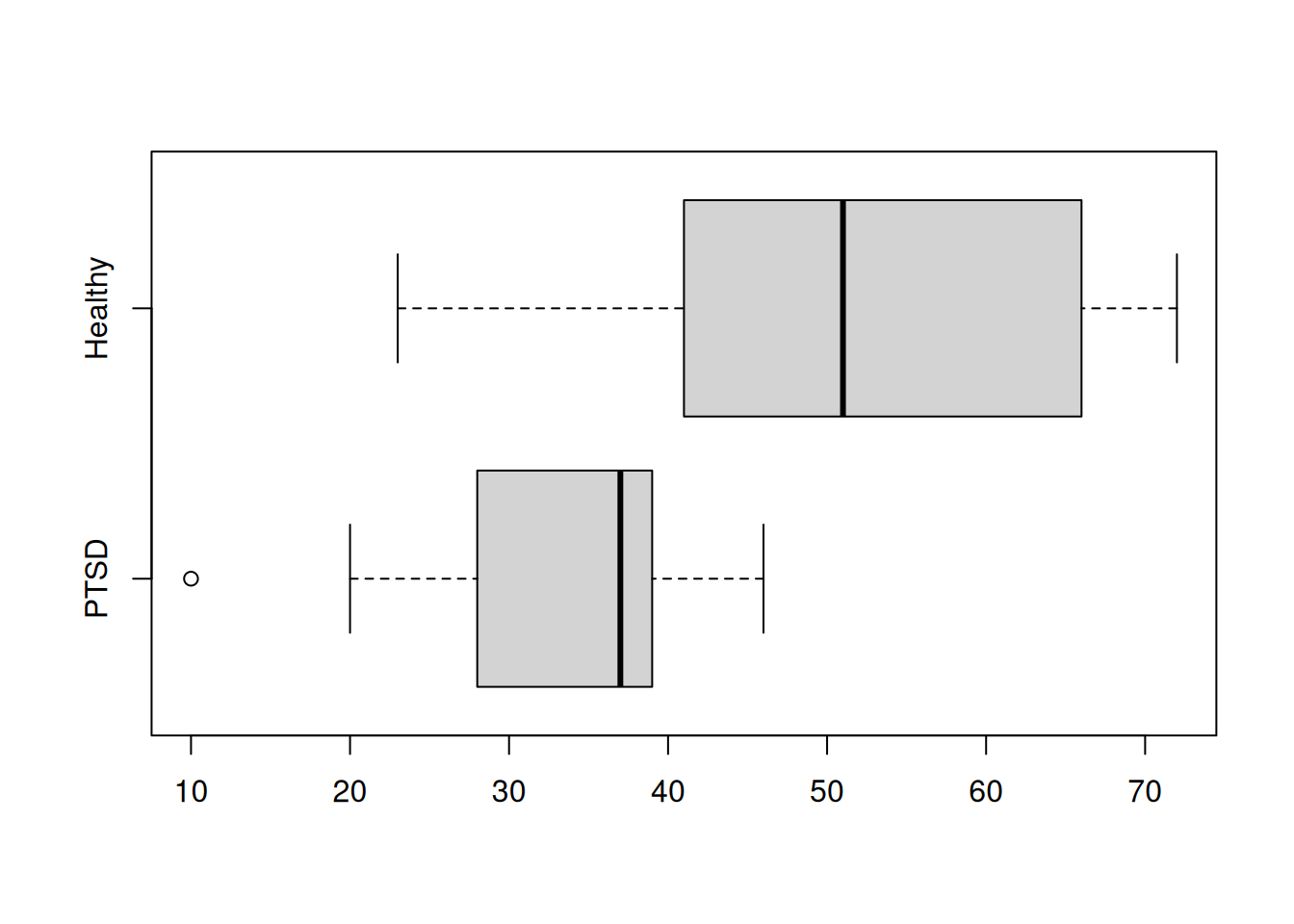# Ch. 1 Descriptive Statistics

Sections covered: all

## 1.1 Populations, Samples, and Processes

To generate a random sample of data, we use the function sample().

Ex: To generate a sample of 6 numbers from the integers between 1 and 20:

sample(x = 1:20, size  = 6)
##  13 20  5  6 17  9

Note: this syntax assumes that there is no replacement (it won’t pick the same number twice). If you want to sample with replacement use the following:

sample(x = 1:20, size = 8, replace = TRUE)
##   8  8 12  1 16  4 12  8

## 1.2 Pictorial and Tabular Methods in Descriptive Statistics

Skip: Example 1.7, p. 15 (double-digit leaves)

Skip: “Dotplots,” pp. 15-16

• Stem-and-leaf display: Ex: The following are the listing prices (in thousands of $) of one-bedroom apartments in Morningside Heights in 2016: 379, 425, 450, 450, 499, 529, 535, 535, 545, 599, 665, 675, 699, 699, 725, 725, 745, 799 Draw a stem an leaf plot of this data: 1. Create a new variable (we’ll cal it “prices”). Note: we always use the syntax <- c() to generate new variables. prices <- c(379, 425, 450, 450, 499, 529, 535, 535, 545, 599, 665, 675, 699, 699, 725, 725, 745, 799) 1. Draw a stem and leaf plot prices <- c(379, 425, 450, 450, 499, 529, 535, 535, 545, 599, 665, 675, 699, 699, 725, 725, 745, 799) stem(prices) ## ## The decimal point is 2 digit(s) to the right of the | ## ## 3 | 8 ## 4 | 355 ## 5 | 03445 ## 6 | 078 ## 7 | 00335 ## 8 | 0 Note that histograms are drawn with unbinned data. R does the binning in the process of drawing the histogram. • Frequency histogram: If you want to create a frequency histogram of this data you use the following: prices <- c(379, 425, 450, 450, 499, 529, 535, 535, 545, 599, 665, 675, 699, 699, 725, 725, 745, 799) hist(prices)Note: histograms are drawn with unbinned data. R does the binning in the process of drawing the histogram. This means that the program chooses the size of the bins for you To add specific bin sizes and colors to your histogram, you can use the following syntax: prices <- c(379, 425, 450, 450, 499, 529, 535, 535, 545, 599, 665, 675, 699, 699, 725, 725, 745, 799) hist(prices, breaks = c(300, 400, 500, 600, 700, 800), col = "lightblue")• Density histogram: We use an almost identical syntax to generate density histograms, but add a condition (freq=FALSE): prices <- c(379, 425, 450, 450, 499, 529, 535, 535, 545, 599, 665, 675, 699, 699, 725, 725, 745, 799) hist(prices, freq = FALSE, breaks = c(300, 400, 500, 600, 700, 800), col = "lightblue", las = 1)Cumulative frequency histogram For this type of histogram, we need access to the bin counts, in order to calculate the cumulative frequencies. The hist() function returns these values, if assigned to a variable: x <- c(1, 1, 1, 1, 1, 5, 5, 5, 7, 7, 8) myhistdata <- hist(x)myhistdata ##$breaks
##  1 2 3 4 5 6 7 8
##
## $counts ##  5 0 0 3 0 2 1 ## ##$density
##  0.45454545 0.00000000 0.00000000 0.27272727 0.00000000 0.18181818 0.09090909
##
## $mids ##  1.5 2.5 3.5 4.5 5.5 6.5 7.5 ## ##$xname
##  "x"
##
## $equidist ##  TRUE ## ## attr(,"class") ##  "histogram" The particular information we want is $counts:

myhistdata$counts ##  5 0 0 3 0 2 1 The cumulative frequencies are: cumsum(myhistdata$counts)
##   5  5  5  8  8 10 11

To plot them, we need to use a bar chart, not a histogram, since we already have the y-axis values:

barplot(cumsum(myhistdata$counts))Cleaned up: barplot(cumsum(myhistdata$counts),
col = "lightblue",
space = 0,  # remove gaps between bars
las = 1, # make all tick mark labels horizontal
ylim = c(0, 12), # make the y-axis longer
names.arg = myhistdata$mids )## 1.3 Measures of location Skip: Example 1.16, p. 33 (trimmed mean) Skip: “Categorical Data and Sample Proportions,” p. 34 (We’ll return to this topic later.) Consider the same dataset (prices). To find the mean, median, quartiles, and trimmed mean, we use the following syntax: prices <- c(379, 425, 450, 450, 499, 529, 535, 535, 545, 599, 665, 675, 699, 699, 725, 725, 745, 799) mean(prices) ##  593.2222 median(prices) ##  572 ## quartiles quantile(prices) ## 0% 25% 50% 75% 100% ## 379.0 506.5 572.0 699.0 799.0 ## trimmed mean mean(prices, trim = .1) ## 10% trimmed mean ##  593.75 ## 1.4 Measures of variability Skip: extreme outliers (p. 42) We will define outliers for boxplots to be observations that are more than 1.5 times the fourth spread from the closest fourth. They may be indicated with either a solid or open circle (in contrast to the book which uses one for mild outliers and the other for extreme outliers.) • Sample variance: prices <- c(379, 425, 450, 450, 499, 529, 535, 535, 545, 599, 665, 675, 699, 699, 725, 725, 745, 799) var(prices) ##  15981.48 • Sample standard deviation: sqrt(var(prices)) ##  126.4179 sd(prices) ##  126.4179 • Five number summary (min, lower-hinge, median, upper-hinge, max) fivenum(prices) ##  379 499 572 699 799 If you want to generate a horizontal boxplot, use the following • Boxplots prices <- c(379, 425, 450, 450, 499, 529, 535, 535, 545, 599, 665, 675, 699, 699, 725, 725, 745, 799) boxplot(prices)boxplot(prices, horizontal = TRUE)(Note: you can also add a color as done below): boxplot(prices, horizontal = TRUE, col='pink')Now, to create a comparative boxplot of two datasets, we will create a “dataframe” (2d array with two dataset values). Then, we will create a boxplot from this “joined” dataset. PTSD <- c(10, 20, 25, 28, 31, 35, 37, 38, 38, 39, 39, 42, 46) Healthy <- c(23, 39, 40, 41, 43, 47, 51, 58, 63, 66, 67, 69, 72) df <- data.frame(Healthy, PTSD) boxplot(df, horizontal = TRUE)## Practice Exercises 1. Using the built-in dataset ToothGrowth in R, visualize the data and comment on the effectiveness of different functions in the context. [Ans] # The first 5 rows of the data head(ToothGrowth, 5) ## len supp dose ## 1 4.2 VC 0.5 ## 2 11.5 VC 0.5 ## 3 7.3 VC 0.5 ## 4 5.8 VC 0.5 ## 5 6.4 VC 0.5 # Five number summary fivenum(ToothGrowth$len)
##   4.20 12.55 19.25 25.35 33.90
# Boxplot
# '$' extracts the column by name boxplot(ToothGrowth$len)# Stem-and-leaf Plot
stem(ToothGrowth$len) ## ## The decimal point is 1 digit(s) to the right of the | ## ## 0 | 4 ## 0 | 5667789 ## 1 | 00001124 ## 1 | 55555677777899 ## 2 | 001222333344 ## 2 | 55566666667779 ## 3 | 0134 # Histogram h <- hist(ToothGrowth$len)# Cumulative Histogram
h$counts <- cumsum(h$counts)
plot(h)### Syntax explanation head(): directly see how the dataset looks; useful when the dataset is large and it’s difficult to display all rows and columns together.

fivenum(): returns the minimum value, lower fourth, median, upper fourth, and maximum value

boxplot(): visualizes the five number summary plus outliers. (It’s clear that the ToothGrowth data is not skewed.)

stem(): compares the number of data points that fall in different bins. (Here we can see that most values are between 20 and 29.)

hist(): draws a histogram – values are grouped in bins

cumsum(): takes a vector and returns the cumulative sums